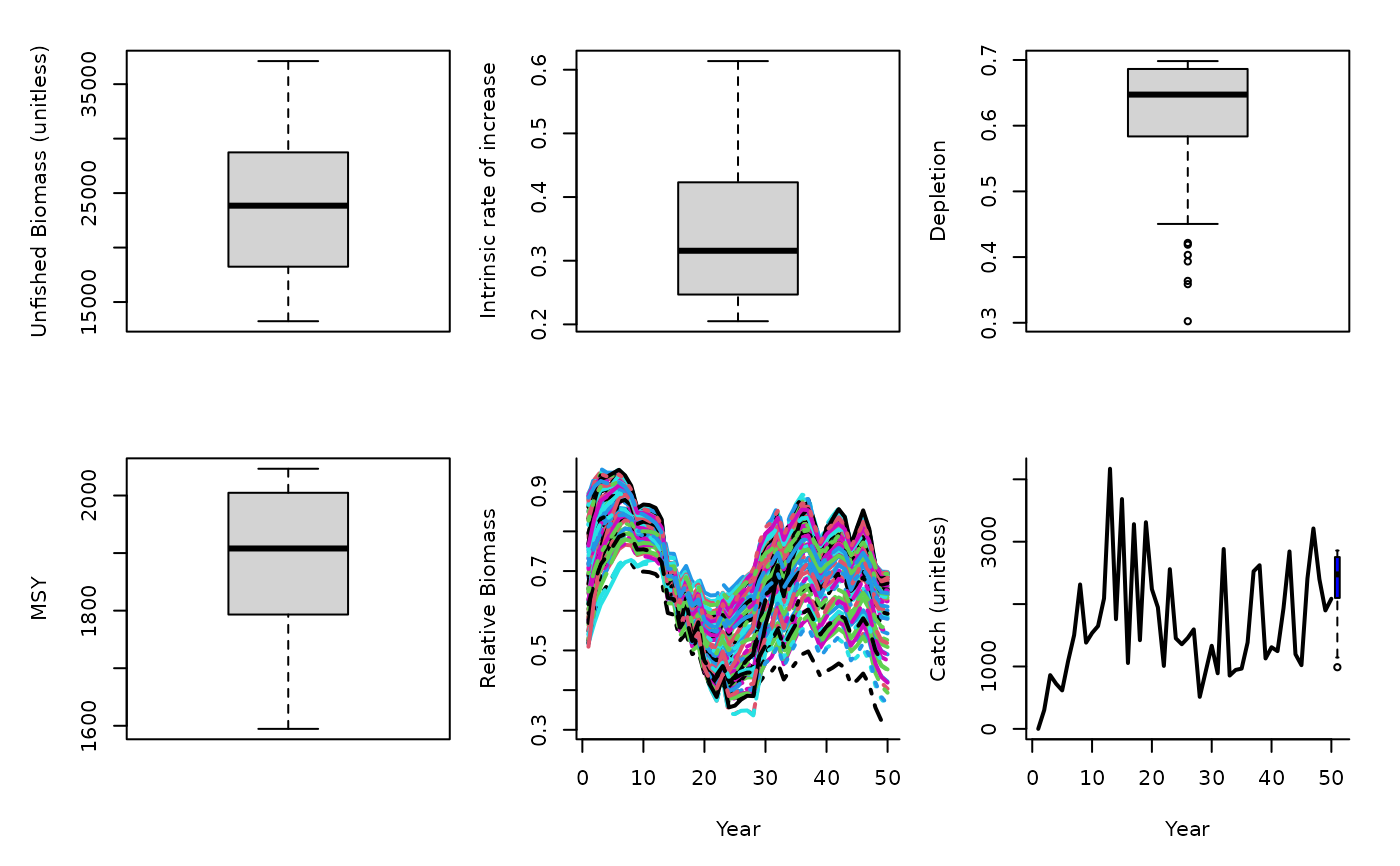An MP that uses Martell and Froese (2012) method for estimating MSY to determine the OFL. Since their approach estimates stock trajectories based on catches and a rule for intrinsic rate of increase it also returns depletion. Given their surplus production model predicts K, r and depletion it is straight forward to calculate the OFL based on the Schaefer productivity curve.

SPMSY(x, Data, reps = 100, plot = FALSE)

## Arguments

x

A position in the data object

Data

A data object

reps

The number of stochastic samples of the MP recommendation(s)

plot

Logical. Show the plot?

## Value

An object of class Rec-class with the TAC slot populated with a numeric vector of length reps

## Details

The TAC is calculated as: $$\textrm{TAC} = D K \frac{r}{2}$$ where $$D$$ is depletion, $$K$$ is unfished biomass, and $$r$$ is intrinsic rate of increasase, all estimated internally by the method based on trends in the catch data and life-history information.

Requires the assumption that catch is proportional to abundance, and a catch time-series from the beginning of exploitation.

Occasionally the rule that limits r and K ranges does not allow r-K pairs to be found that lead to the depletion inferred by the catch trajectories. In this case this method widens the search.

## Required Data

See Data-class for information on the Data object

SPMSY: Cat, L50, MaxAge, vbK, vbLinf, vbt0

## Rendered Equations

See Online Documentation for correctly rendered equations

Other Surplus production MPs: Fadapt(), Rcontrol(), SPSRA(), SPmod(), SPslope()

T. Carruthers

## Examples

SPMSY(1, Data=MSEtool::SimulatedData, plot=TRUE)#> TAC (median)
#>     2480.489